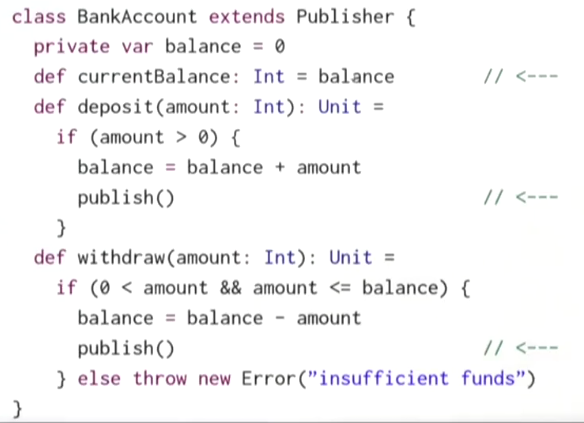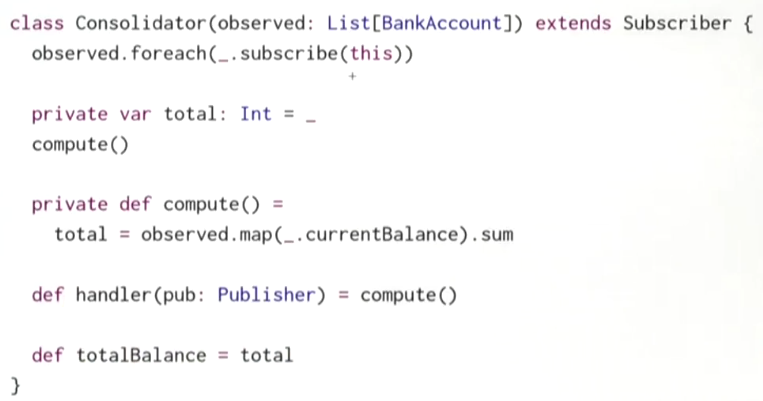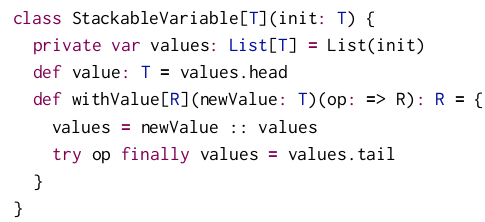# [Scala MOOC II] Lec4 - Timely Effects

[TOC]

this week: discuss how to handle events in user-interface — MVC, functional reactive programming.

# Lecture 4.1 - Imperative Event Handling: The Observer Pattern

Traditional way of handling events: observer Pattern (MVC). Used when views need to react to change in a model.

MVC: model-view-controller for user interface

• Views can announce themselves to a model (called "substribe")
• Models can "publish" new informations to views
```trait Publisher{
private var subscribers: Set[Subscriber] = Set()
def subscribe(subscriber: Subscriber): Unit
def unsubscribe(subscriber: Subscriber): Unit
def publish(): Unit = subscribers.foreach(_.handler(this))
}
trait Substriber{
def handler(pub: Publisher)
}
```

make the `BankAccount` a `Publisher`:create a `Consolidator` that displays bank accounts:Advantages of MVC:

• decouples views from stat
• have varying number of views of a given state
• simple to set up

Disadvantages:

• forces imperative style since handlers are of Unit type
• many moving parts that need to be coordinated
• concurrency will be more complicated (ex. 2 models update one view at the same time)
• Views are tightly bound to one state, view updates immediately

# Lecture 4.2 - Functional Reactive Programming

FRP:

• reactive programming: react to seq of events that happen in time.
• functional view: aggregate an event sequence to a signal.

In our simple API, the most important concept is `Signal`.

Signal:

• is a vlaue that changes over time
• represented as a function mapping time to value domain
• define new signals from existing ones (instead of having mutable state)

example: move mouse positions

event-based view:
whenever mouse moves, an event `MouseMoved(toPos: Position)` is fired

FRP view:
use a signal(function): `mousePosition: Signal[Position]` , which at any point represents a current mouse position

### Signal opeartions

2 fundamental ops on signals:

• obtain value of signal at current time (the `apply` function): `mousePosition()`
• define a signal in term of another signal (constructor `Signal(expr)`)

example: from the mouse curve signal, define a new signal indicating whether the curve is inside the rectangle or not.

```def inReactangle(LL: Position, UR: Position): Signal[Boolean] =
Signal{val pos = mousePosition() // the mouse pos signal
LL<= pos && pos <= UR}
```

use `Signal(_value)` to define a constant signal:

`val sig = Signal(3) // constant signal`

then define a subclass `Var` of `Signal` for changable signals, which has an `update` operation to redefine the value of a signal.

```val sig = Var(3)
sig.update(5) // update
```

⇒ In scala, `update` is also a special function: assignments like `a(e1,...en)=e` are translated to `a.update(e1...en, e)`. (Here the n could be 0, i.e. no arguments in the assignment expression).

→ So `sig.update(5)` can be re-written as `sig()=5`. The `()` is like dereferencing a varable.

Difference between `Var`s and mutable `var`s: we can map over signals, i.e. maintain a relation between 2 signals forever in the future, whereas using mutable `var`s have to propagate all updates manually.

### example: bank account

add a signal `balance` to `BankAccount`s, define a function `consolidated` which takes sum of all balances of accounts in list.

```class BankAccount {
val balance = Var(0) // a Var signal
def deposit(amount: Int): Unit = if(amount>0){
val b = balance()
balance() = b + amount // otherwise cyclic definition of `balance`
}
def withdraw(amount: Int):Unit =
if(0<amount && amount<=balance()){
val b = balance()
balance() = b - amount
}else throw new Error("insufficient balance")
}

def consolidated(accts: List[BankAccount]):Signal[Int] =
Signal(accts.map(_.balance()).sum)

// similarly, define exange rate signals
def xchange = Signal(246.0)
def inDollar = Signal(c()*xchange)
```

note the difference between var assignment and signal update:

• `v = v+1`: the new value is old value + 1
• `s() = s() + 1`: the s is a function that is always 1 larger than itself (cyclic definitions)

# Lecture 4.3 - A Simple FRP Implementation

implementation of `Singal` and `Var`.

class API:

```class Signal[T](expr: =>T){
def apply(): T = ??? // s() give cur value
}

object Signal{
def apply[T](expr: =>T) = new Signal(expr) // construct new signal
}

class Var[T](expr: =>T) extends Signal[T](expr){
def update(expr: =>T):Unit = ??? // s()=expr for update
}

object Var{
def apply[T](expr: =>T) = new Var(expr)
}
```

or more convientently:

• use `sig()` get the signal's (current) value
• use `sig() = {new_expr}` to update the signal's expression

## implemention idea

### Signal

each `sig: Signal[T]` maintains:

• its *current value *

`private var myValue: T`

• its *current expression *

`private var myexpr: () => T`

• set of observers : other signals (callersigs) that depend on `this.myValue` — if `this.myValue` changes, all signals in this.observers should be re-evaluated

`private var observers: Set[Signal[_]]`

• protected function to re-evaluate value

`protected def computeValue(): Unit`

• protected function to change expression

`protected def update(expr: => T): Unit`

How to record dependencies:

• when evaluating a `Signal`, need to know which `callersig` gets defined by `this`
• So we should add the caller to `this.observers` when `apply` is called (like: `sig()`).
• if `this.myValue` changes (when calling `computeValue()`), all caller signals in `this.observers` are re-evaluated (`callersig.computeValue()`); and `this.observers` is cleared(!! see next item).
• when `callersig`s re-evaluate their expression, the `apply()` method will add the `callersig` again to `this.observers`

### caller

How to find out who is calling so that a signal is evaluated ? simplistic way: maintain a global data structure (stack-fashion) referring to current `caller: StackableVariable[Signal[T]]`.

`caller` is a global "stack" of `callersig`s that get poped/pushed.

The API of the `StackableVariable[Signal[T]]` class:

• `caller.value: Signal[T]`:

get the callersig on top of stack, which depends on currently evaluating signal (`this`), and so should be added to `this.observers`.

• `caller.withValue(sig:Signal[T])(expr: () => [T])`:

first add `sig` to the top of stack; then evaluate `expr`; finally pop `sig` off the stack.

Here is the implementation of the `caller` :• So whenever `sig` want to know who depends on it, it just use `caller.value`;

thus, in the `apply` method of `Signal`s, we write like this:

```def apply() = {
observers += caller.value // caller.value=top of stack, it depends on currenlty-evaluating value (this), so it should be added to this.observers
assert(!caller.value.observers.contains(this), "cyclic signal definition")
myValue
}
```
• And if `sig` want to depend on other signals, in order to write the expression(which includes other signals that sig depends on), it use: `caller.withValue(this){expr...}`

so in `computeValue()`, as `this.myExpr` may contain other signals that `this` depend on, we should write:

```protected def computeValue(): Unit = {
for (sig <- observed)
sig.observers -= this
observed = Nil
val newValue = caller.withValue(this)(myExpr()) // withValue will add this to the top of stack, so when eval other signals, they know that it's this signal that depends on them
if (myValue != newValue) { // re-evaluate all callersigs that depends on this
myValue = newValue
val obs = observers
observers = Set() // clear observers for this: the callersigs may be added back in apply()
obs.foreach(_.computeValue()) // here this.observers might be added with calersigs
}
}
```

problem: global stack is not good... especially for concurrency ⇒ replace global state by thread-local state.

Or use implicit parameteres: pass current value of the thread-local variable into a signal expr as implicit parameter.

# Lecture 4.4/4.5 - Latency as an Effect

(I didn't quite get the point from this lecture on...)

when computation takes a lot of time: register a callback when computation terminates (either success or failure).

`Future[T]`: a monad that handles both exceptions and latency

```trait Future[T] {
def onComplete(callback: Try[T] =>Unit): Unit
}
```

The callback use pattern matching:

```ts match{
case Success(t) => onNext(t)
case Failure(e) => onError(e)
}
```

another option: give 2 callbacks, one for success, one for failure.

`def onCompelet(success: T=>Unit, failed: Throwable => Unit): Unit`

# Lecture 4.6/4.7/4.8/4.9/4.10 - Combinators on Futures/Composing Futures

higher-order funcitons on `Future`s: map/filter/flatMap/...

recover/recoverWith for Error case ⇔ map/flatMap for Future.

fallbackTo:

retry: deal with failure...

turn recursion to foldleft/foldright...# Conclusion

• lazy evaluation: infinite data structure
• distinction between computations and values: random/signal are computations
• monads: abstract over properties of computations, encapsulate mutations, ...

mix FP and mutable state

• laziness
• FRP
• monads# exercice: calculator

Use Function Reactive Programming (FRP), with the Signal[A] class that you have seen in the lectures, to implement a spreadsheet-like calculator. In this calculator, cells can depend on the value of other cells, and are recomputed automatically when the latter change.

#### Part 4 of series «Functional Program Design in Scala»：

comments powered by Disqus